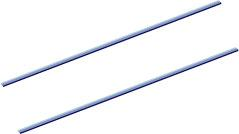# (instructions: 3314

Find the distance of the parallels, which equations are:
x = 3-4t, y = 2 + t and x = -4t, y = 1 + t
(instructions: select a point on one line and find its distance from the other line)

x =  1.6977

### Step-by-step explanation:Did you find an error or inaccuracy? Feel free to write us. Thank you!

Showing 1 comment:
Math student
Using a ruler a pair of compasses only construct triangle ABC in which AB=5cm BC=5.9cm and<BAC=45°

Tips for related online calculators
The line slope calculator is helpful for basic calculations in analytic geometry. The coordinates of two points in the plane calculate slope, normal and parametric line equation(s), slope, directional angle, direction vector, the length of the segment, intersections of the coordinate axes, etc.
Our vector sum calculator can add two vectors given by their magnitudes and by included angle.